# Non/Not Mutually exclusive

Can both happen (at the same time)

Mutually exclusive - Time - Can't both happen
(at the same time) (see previous definition)

So Non/Not Mutually Exclusive - Time - CAN
both happen at the same time

Non/not mutually exclusive is the opposite to mutually exclusive.

Example

If a die is rolled find the probability of rolling a number less than 3 or an even number.

Remember\ probability\=(N\umber\ of\ RIGHT\ answers)/(N\umber\ of \ALL\ possibl\e\ answers

Start by drawing a probability tree for a roll of the dice that gives less than 3: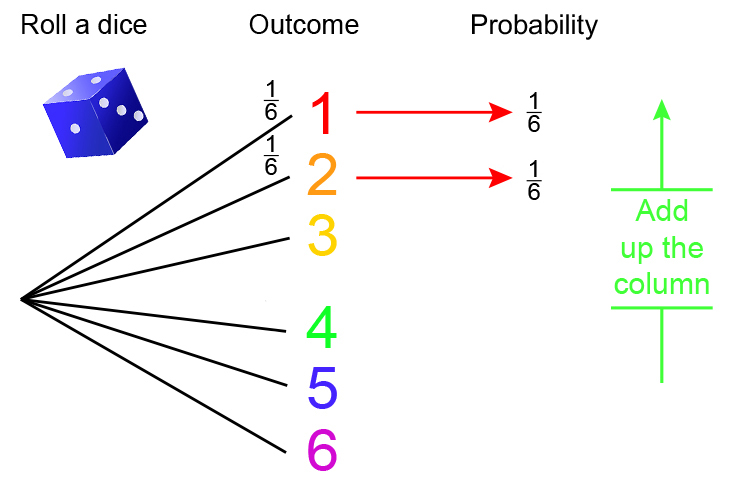1/6+1/6=2/6

Now draw the probability tree for even numbers: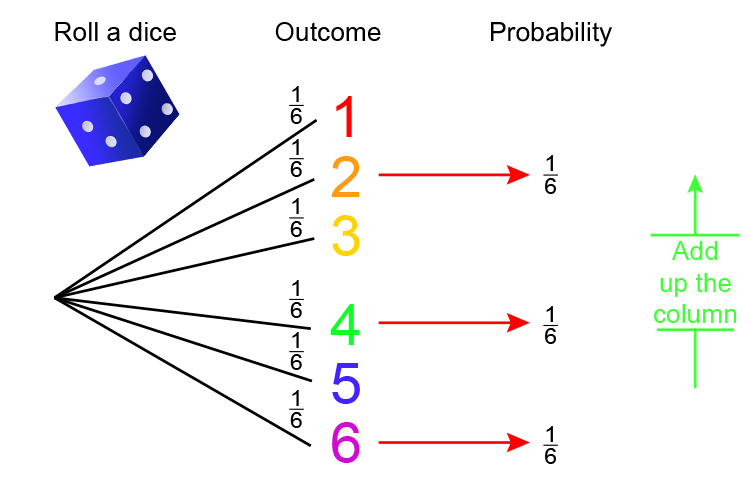1/6+1/6+1/6=3/6

So  2/6+3/6=5/6

But note the probability of getting 2 comes up twice, i.e.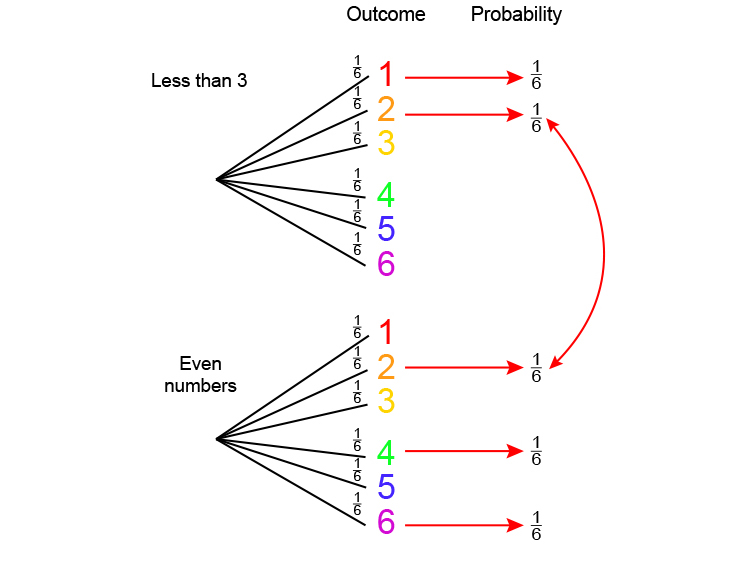A Venn diagram shows the problem: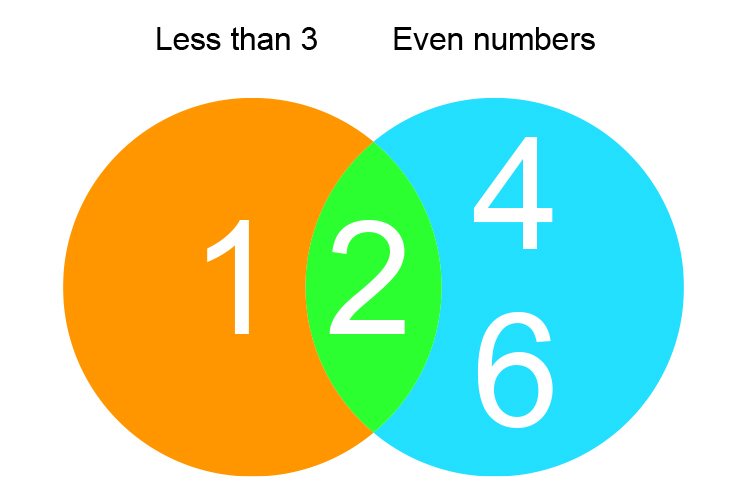In these cases you have to remove one of the crossovers to get the answer.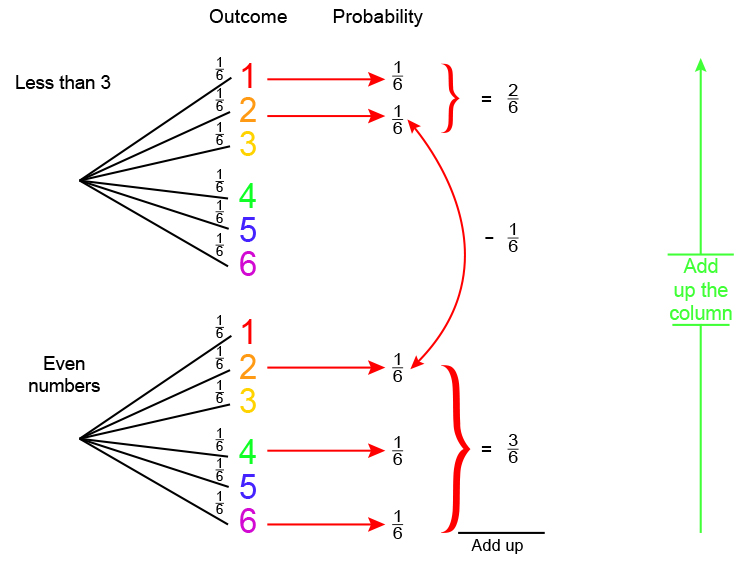Add up 2/6+3/6  but remove 1/6

2/6+3/6=5/6

5/6-1/6=4/6

4/6=2/3

The probability of rolling a number less than 3 or an even number is 2 in 3 or 0.67.

And if 1 = 100% then 0.67 = 67% chance.

Example 2

What is the probability of you picking an ace or a heart from a pack of cards?

Remember\ probability\=(N\umber\ of\ RIGHT\ answers)/(N\umber\ of \ALL\ possibl\e\ answers

For 1 card probability = 1/52

Start by drawing a probability tree:

## For Aces: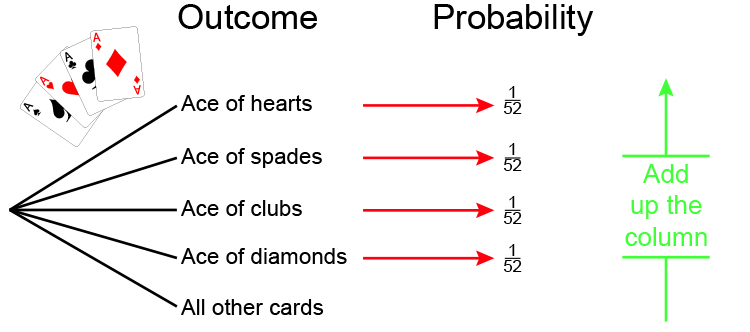1/52+1/52+1/52+1/52=4/52

## For Hearts: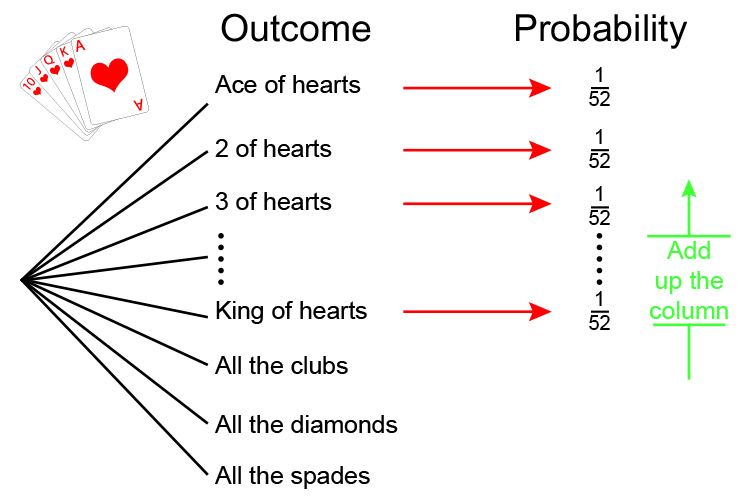1/52+1/52+1/52+1/52+1/52+1/52+1/52+1/52+1/52+1/52+1/52+1/52+1/52=13/52

But note the ace of hearts is in both.

So remove one of the ace of hearts.

So total = 4/52+13/52-1/52

So total = (4+13-1)/52=16/52

16/52=8/26=4/13